# Introduction to GPy: Gaussian Process Regression in GPy¶

## Gaussian Process Winter School, Genova, Italy¶

### Neil D. Lawrence and Nicolas Durrande¶

In :
%matplotlib inline
import numpy as np
import pods
import pylab as plt
import GPy
from IPython.display import display

/Users/neil/Library/Enthought/Canopy_64bit/User/lib/python2.7/site-packages/pytz/__init__.py:29: UserWarning: Module pods was already imported from /Users/neil/sods/ods/pods/__init__.pyc, but /Users/neil/Library/Enthought/Canopy_64bit/User/lib/python2.7/site-packages is being added to sys.path
from pkg_resources import resource_stream


## Covariance Function Parameter Estimation¶

In this session we are going to optimize the parameters of the Gaussian process using gradient based optimization approaches. These maximize the likelihood function: which is defined as the probability of the model given the parameters, $p(\mathbf{y}|\mathbf{X}, \boldsymbol{\theta})$.

First we'll load in the olympic marathon data.

In :
data = pods.datasets.olympic_marathon_men()
x = data['X']
y = data['Y']


Then we'll construct a Gaussian process model with an exponentiated quadratic covariance function.

In :
k = GPy.kern.RBF(1)
model = GPy.models.GPRegression(x, y, k)
display(model)
model.plot()


Model: GP regression
Log-likelihood: -118.821194703
Number of Parameters: 3

GP_regression. Value Constraint Prior Tied to
rbf.variance 1.0 +ve
rbf.lengthscale 1.0 +ve
Gaussian_noise.variance 1.0 +ve
Out:
{'dataplot': [<matplotlib.lines.Line2D at 0x10ecb5990>],
'gpplot': [[<matplotlib.lines.Line2D at 0x10ecb2550>],
[<matplotlib.patches.Polygon at 0x10ecb2790>],
[<matplotlib.lines.Line2D at 0x10ecb2dd0>],
[<matplotlib.lines.Line2D at 0x10ecb5350>]]}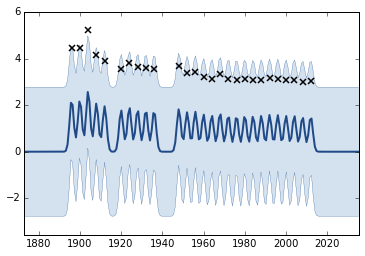Rather sensibly, we've given the model an initial plot, and we can clearly see that the inital length scale is too low. This makes sense, our prior says that the length scale is 1 year, which means that athletic performance varies across very short time scales. This is perhaps unlikely, let's choose a larger lengthscale and try again.

In :
model.rbf.lengthscale = 10.
model.plot()

Out:
{'dataplot': [<matplotlib.lines.Line2D at 0x10f27aed0>],
'gpplot': [[<matplotlib.lines.Line2D at 0x10f277a90>],
[<matplotlib.patches.Polygon at 0x10f277cd0>],
[<matplotlib.lines.Line2D at 0x10f27a350>],
[<matplotlib.lines.Line2D at 0x10f27a890>]]}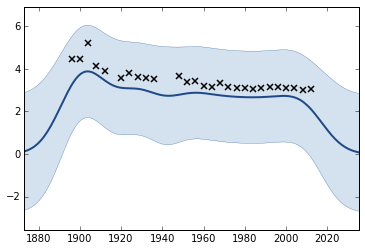That seems better, now we can think about optimization. First though we have to consider the fact that some of the parameters are constrained (for example lengthscales and variances can only be positive). GPy allows the user to specify such constraints when constructing the model.

### Parameter Constraints¶

As we have seen during the lectures, the parameters values can be estimated by maximizing the likelihood of the observations. Since we don’t want one of the variance to become negative during the optimization, we can constrain all parameters to be positive before running the optimisation.

In :
model.constrain_positive('.*') # Constrains all parameters matching .* to be positive, i.e. everything

WARNING: reconstraining parameters GP_regression


The warnings are because the parameters are already constrained by default, the software is warning us that they are being reconstrained.

Now we can optimize the model using the model.optimize() method.

In :
model.optimize()
model.plot()
display(model)


Model: GP regression
Log-likelihood: -6.94713791215
Number of Parameters: 3

GP_regression. Value Constraint Prior Tied to
rbf.variance 25.3995048241 +ve
rbf.lengthscale 152.045313 +ve
Gaussian_noise.variance0.048506484546 +ve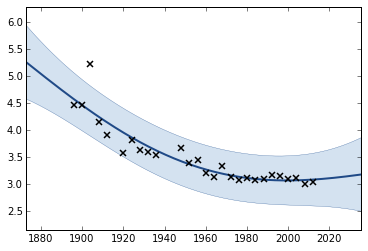The parameters obtained after optimisation can be compared with the values selected by hand above. As previously, you can modify the kernel used for building the model to investigate its influence on the model.

By adding covariance functions together we can try and decompose the observation in to a longer lengthscale process and a shorter lengthscale process. Below we consider a GP that is initialised with a long lengthscale exponentiated quadratic, and a Matern $\frac{5}{2}$ covariance to take account of shorter lengthscale effects. We also add a bias term to allow for an overall average.

In :
# Exercise 5 a) answer
kern = GPy.kern.RBF(1, lengthscale=80) + GPy.kern.Matern52(1, lengthscale=10) + GPy.kern.Bias(1)
model = GPy.models.GPRegression(x, y, kern)
model.optimize()
model.log_likelihood()
display(model)


Model: GP regression
Log-likelihood: -5.99078279431
Number of Parameters: 6

GP_regression. Value Constraint Prior Tied to
Gaussian_noise.variance0.0368133074589 +ve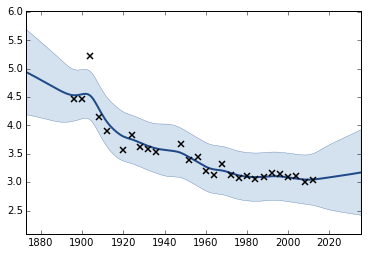### Exercise 2¶

Now model Model the data with a product of an exponentiated quadratic covariance function and a linear covariance function. Fit the covariance function parameters. Why are the variance parameters of the linear part so small? How could this be fixed?

In :
# Exercise 2 answer


# DRAFT¶

## Gene Expression Example¶

We now look at a real data example where there are multiple modes to the solution. In Kalaitzis and Lawrence the objective was to understand when a temporal gene expression was either noise or had some underlying signal. To determine this Gaussian process models were fitted with and without a temporal kernel, and the likelihoods were compared. In the thousands of genes they considered, there were some where the posterior error surface for the lengthscale and the signal/noise ratio was multi modal. We will consider one of those genes. The example can also be rerun as

GPy.examples.regression.multiple_optima()


The first thing to do is write a helper function for computint the likelihoods add different signal/noise ratios and different lengthscales. This is to allow us to visualize the error surface.

In :
def contour_objective(x, y, log_length_scales, log_SNRs, kernel_call=GPy.kern.RBF):
'''Helper function to contour an objective function in a set up where there
is a kernel for signal corrupted by noise.'''
lls = []
num_data=y.shape
kernel = kernel_call(1, variance=1., lengthscale=1.)
model = GPy.models.GPRegression(x, y, kernel=kernel)
y = y - y.mean()
for log_SNR in log_SNRs:
SNR = 10.**log_SNR
length_scale_lls = []
for log_length_scale in log_length_scales:
model['.*lengthscale'] = 10.**log_length_scale
model.kern['.*variance'] = SNR
Kinv = GPy.util.linalg.pdinv(model.kern.K(x)+np.eye(num_data))
total_var = 1./num_data*np.dot(np.dot(y.T, Kinv), y)
noise_var = total_var
signal_var = SNR*total_var
model.kern['.*variance'] = signal_var
model.Gaussian_noise = noise_var
length_scale_lls.append(model.log_likelihood())
print SNR, 10.**log_length_scale
display(model)
lls.append(length_scale_lls)

return np.array(lls)


Now we load in the data and compute the likelihood values.

In :
data = pods.datasets.della_gatta_TRP63_gene_expression(gene_number=937)
x = data['X']
y = data['Y']
y = y - y.mean()
kern = GPy.kern.RBF(input_dim=1)
model = GPy.models.GPRegression(x, y, kern)
resolution = 2
log_lengthscales = np.linspace(1, 3.5, resolution)
log_SNRs = np.linspace(-2.5, 1., resolution)
lls = contour_objective(x, y, log_lengthscales, log_SNRs)

0.00316227766017 10.0


Model: GP regression
Log-likelihood: -2.10275061954
Number of Parameters: 3

GP_regression. Value Constraint Prior Tied to
rbf.variance 0.00025506380619 +ve
rbf.lengthscale 10.0 +ve
Gaussian_noise.variance 0.0806582576232 +ve
0.00316227766017 3162.27766017


Model: GP regression
Log-likelihood: -2.12547857371
Number of Parameters: 3

GP_regression. Value Constraint Prior Tied to
rbf.variance 0.000255972082804 +ve
rbf.lengthscale 3162.27766017 +ve
Gaussian_noise.variance 0.0809454799079 +ve
10.0 10.0


Model: GP regression
Log-likelihood: -1.41450534969
Number of Parameters: 3

GP_regression. Value Constraint Prior Tied to
rbf.variance 0.0671183959588 +ve
rbf.lengthscale 10.0 +ve
Gaussian_noise.variance0.00671183959588 +ve
10.0 3162.27766017


Model: GP regression
Log-likelihood: -4.33641210151
Number of Parameters: 3

GP_regression. Value Constraint Prior Tied to
rbf.variance 0.779949847367 +ve
rbf.lengthscale 3162.27766017 +ve
Gaussian_noise.variance0.0779949847367 +ve
In :
display(model)


Model: GP regression
Log-likelihood: -16.7147336689
Number of Parameters: 3

GP_regression. Value Constraint Prior Tied to
rbf.variance 1.0 +ve
rbf.lengthscale 1.0 +ve
Gaussian_noise.variance 1.0 +ve
In :
plt.contour(lengthscales, log_SNRs, lls, 20, cmap=plt.cm.jet)

---------------------------------------------------------------------------
NameError                                 Traceback (most recent call last)
<ipython-input-37-aaa47ce1d09d> in <module>()
1
----> 2 plt.contour(lengthscales, log_SNRs, lls, 20, cmap=plt.cm.jet)

NameError: name 'lengthscales' is not defined
In :
plt.plot(x, y-y.mean())

Out:
[<matplotlib.lines.Line2D at 0x1121cc1d0>]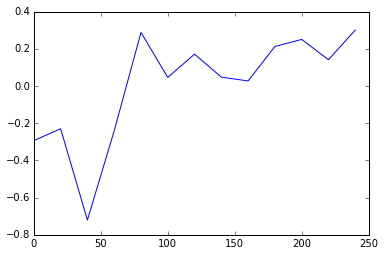### Sampling with Hamiltonian Monte Carlo¶

In :
model.kern.lengthscale=30.
model.kern.variance=0.5
model.Gaussian_noise=0.01
model.kern.lengthscale.set_prior(GPy.priors.InverseGamma.from_EV(30.,100.))
model.kern.variance.set_prior(GPy.priors.InverseGamma.from_EV(0.5, 1.)) #Gamma.from_EV(1.,10.))
model.Gaussian_noise.set_prior(GPy.priors.InverseGamma.from_EV(0.01,1.))
_ = model.plot()

WARNING: reconstraining parameters GP_regression.rbf.lengthscale
WARNING: reconstraining parameters GP_regression.rbf.lengthscale
WARNING: reconstraining parameters GP_regression.rbf.variance
WARNING: reconstraining parameters GP_regression.rbf.variance
WARNING: reconstraining parameters GP_regression.Gaussian_noise
WARNING: reconstraining parameters GP_regression.Gaussian_noise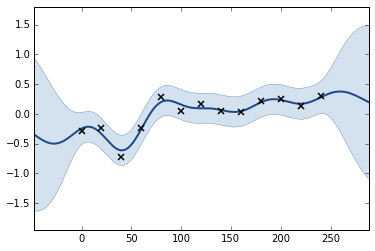In :
hmc = GPy.inference.mcmc.HMC(model, stepsize=5e-2)
s = hmc.sample(num_samples=1000)

In :
plt.plot(s)

Out:
[<matplotlib.lines.Line2D at 0x112116250>,
<matplotlib.lines.Line2D at 0x1121bf350>,
<matplotlib.lines.Line2D at 0x1121bf8d0>]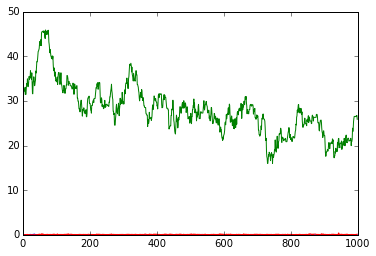In :
labels = ['kern variance', 'kern lengthscale','noise variance']
samples = s[300:] # cut out the burn-in period
from scipy import stats
xmin = samples.min()
xmax = samples.max()
xs = np.linspace(xmin,xmax,100)
for i in xrange(samples.shape-1):
kernel = stats.gaussian_kde(samples[:,i])
plt.plot(xs,kernel(xs),label=labels[i])
_ = plt.legend()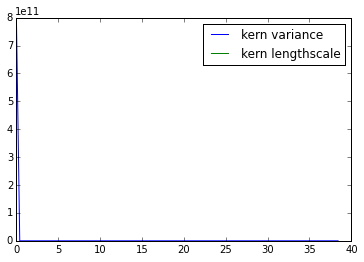In :
fig = plt.figure(figsize=(14,4))
_=ax.plot(samples[:,0],samples[:,1],'.')
ax.set_xlabel(labels); ax.set_ylabel(labels)
_=ax.plot(samples[:,1],samples[:,2],'.')
ax.set_xlabel(labels); ax.set_ylabel(labels)
_=ax.plot(samples[:,0],samples[:,2],'.')
ax.set_xlabel(labels); ax.set_ylabel(labels)

Out:
<matplotlib.text.Text at 0x111d6d490>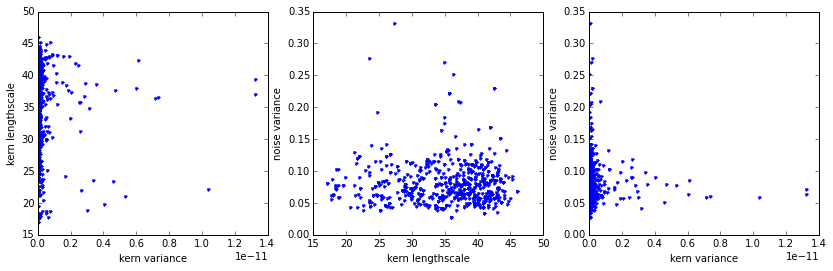Let $x$ be a random variable defined over the real numbers, $\Re$, and $f(\cdot)$ be a function mapping between the real numbers $\Re \rightarrow \Re$. Uncertainty propagation is the study of the distribution of the random variable $f ( x )$.

We will see in this section the advantage of using a model when only a few observations of $f$ are available. We consider here the 2-dimensional Branin test function defined over [−5, 10] × [0, 15] and a set of 25 observations as seen in Figure 3.

In :
# Definition of the Branin test function
def branin(X):
y = (X[:,1]-5.1/(4*np.pi**2)*X[:,0]**2+5*X[:,0]/np.pi-6)**2
y += 10*(1-1/(8*np.pi))*np.cos(X[:,0])+10
return(y)

# Training set defined as a 5*5 grid:
xg1 = np.linspace(-5,10,5)
xg2 = np.linspace(0,15,5)
X = np.zeros((xg1.size * xg2.size,2))
for i,x1 in enumerate(xg1):
for j,x2 in enumerate(xg2):
X[i+xg1.size*j,:] = [x1,x2]

Y = branin(X)[:,None]


We assume here that we are interested in the distribution of $f (U )$ where $U$ is a random variable with uniform distribution over the input space of $f$. We will focus on the computation of two quantities: $E[ f (U )]$ and $P( f (U ) > 200)$.

## Computation of $E[f(U)]$¶

The expectation of $f (U )$ is given by $\int_x f ( x )\text{d}x$. A basic approach to approximate this integral is to compute the mean of the 25 observations: np.mean(Y). Since the points are distributed on a grid, this can be seen as the approximation of the integral by a rough Riemann sum. The result can be compared with the actual mean of the Branin function which is 54.31.

Alternatively, we can fit a GP model and compute the integral of the best predictor by Monte Carlo sampling:

In :
# Fit a GP
# Create an exponentiated quadratic plus bias covariance function
kg = GPy.kern.RBF(input_dim=2, ARD=True)
kb = GPy.kern.Bias(input_dim=2)
k = kg + kb

# Build a GP model
model = GPy.models.GPRegression(X,Y,k)

# fix the noise variance to something low
model.Gaussian_noise.variance = 1e-5
model.Gaussian_noise.variance.constrain_fixed()
display(model)

# optimize the model
model.optimize()

# Plot the resulting approximation to Brainin
# Here you get a two-d plot becaue the function is two dimensional.
model.plot()

# Compute the mean of model prediction on 1e5 Monte Carlo samples
Xp = np.random.uniform(size=(1e5,2))
Xp[:,0] = Xp[:,0]*15-5
Xp[:,1] = Xp[:,1]*15
Yp = model.predict(Xp)
np.mean(Yp)

WARNING: reconstraining parameters GP_regression.Gaussian_noise.variance


Model: GP regression
Log-likelihood: -74285.8774977
Number of Parameters: 5

GP_regression. Value Constraint Prior Tied to
Gaussian_noise.variance1e-05 fixed
Index GP_regression.add.rbf.lengthscale Constraint Prior Tied to
28.9811652911+veN/A
303.000201018+veN/A
Out:
58.283419091796873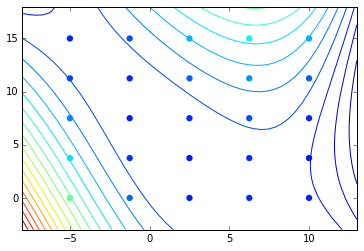### Exercise 6¶

a) Has the approximation of the mean been improved by using the GP model?

b) One particular feature of GPs we have not use for now is their prediction variance. Can you use it to define some confidence intervals around the previous result?

In [ ]:
# Exercise 6 b) answer


## Computation of $P( f (U ) > 200)$¶

In various cases it is interesting to look at the probability that $f$ is greater than a given threshold. For example, assume that $f$ is the response of a physical model representing the maximum constraint in a structure depending on some parameters of the system such as Young’s modulus of the material (say $Y$) and the force applied on the structure (say $F$). If the later are uncertain, the probability of failure of the structure is given by $P( f (Y, F ) > \text{f_max} )$ where $f_\text{max}$ is the maximum acceptable constraint.

### Exercise 7¶

a) As previously, use the 25 observations to compute a rough estimate of the probability that $f (U ) > 200$.

In [ ]:
# Exercise 7 a) answer


b) Compute the probability that the best predictor is greater than the threshold.

In [ ]:
# Exercise 7 b) answer


c) Compute some confidence intervals for the previous result

In [ ]:
# Exercise 7 c) answer


These two values can be compared with the actual value {$P( f (U ) > 200) = 1.23\times 10^{−2}$ .

We now assume that we have an extra budget of 10 evaluations of f and we want to use these new evaluations to improve the accuracy of the previous result.

### Exercise 8¶

a) Given the previous GP model, where is it interesting to add the new observations if we want to improve the accuracy of the estimator and reduce its variance?

b) Can you think about (and implement!) a procedure that updates sequentially the model with new points in order to improve the estimation of $P( f (U ) > 200)$?
# Exercise 8 b) answer